### AmbiguitYtiugibmA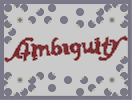Hover over the thumbnail for a full-size version.

Author schmidty563 author:schmidty563 n-art rated 2006-03-20 4 by 54 people. \$AmbiguitYtiugibmA#schmidty563#none#;<6=;<00;<0000000;<:9;<:=006=7<:90000000:900:=800000:=000000000000007=007800000000000007800:;806900000000000006907<:=0000000000000000000:=000;800000000000007<;<0000:=0000000000000:=:9007<00000000000000;800000:=00000000000000:=0000000;<000000000000000000;<0:9000000000000;<0;<0:=0000000000000006=0:=000000000000000000000000000;<000000000000000000000:=0000000000000;<000000000000000000000:=000000000000000000000000000;<0;8000000000000000;<0:=0:=0000000000007<0:=000000000000000000:=0000000;<00000000000000;<000006=00000000000000:9007<;<0000000000000;<0000:=:90000000000000:9000;<0000000000000000000;<:907800000000000007806=<006900000000000006900;900000000000000;<000006;<007<00000007<:9;800;<:=7<:=0000000:=00:=;8:=|12^30,348!12^30,354!12^30,360!12^36,348!12^42,348!12^36,366!12^42,372!12^48,366!12^54,366!12^60,360!12^66,360!12^72,354!12^78,348!12^84,342!12^90,336!12^96,336!12^102,342!12^108,348!12^114,348!12^120,348!12^126,348!12^132,348!12^132,342!12^132,336!12^126,336!12^120,336!12^114,336!12^114,330!12^114,324!12^126,324!12^132,324!12^138,324!12^144,324!12^144,330!12^144,336!12^144,342!12^144,348!12^144,354!12^144,360!12^144,366!12^150,360!12^156,360!12^156,354!12^156,348!12^156,342!12^162,336!12^168,330!12^174,324!12^180,324!12^180,318!12^180,312!12^174,312!12^168,312!12^168,306!12^168,300!12^168,294!12^168,288!12^168,282!12^168,276!12^168,270!12^168,264!12^162,258!12^156,258!12^150,258!12^150,252!12^150,246!12^150,240!12^156,240!12^162,240!12^168,240!12^174,240!12^180,240!12^186,246!12^192,246!12^198,240!12^198,234!12^192,228!12^186,228!12^180,228!12^174,228!12^168,228!12^162,228!12^156,228!12^150,234!12^144,240!12^138,240!12^132,246!12^126,252!12^120,258!12^120,264!12^114,270!12^108,276!12^108,282!12^102,288!12^96,294!12^96,300!12^90,306!12^90,312!12^84,318!12^84,324!12^78,330!12^78,336!12^72,342!12^66,348!12^60,354!12^54,354!12^48,354!12^42,354!12^36,354!12^36,360!12^42,360!12^48,360!12^54,360!12^42,366!12^66,354!12^72,348!12^78,342!12^84,336!12^84,330!12^96,330!12^90,330!12^102,330!12^108,330!12^102,336!12^108,336!12^108,342!12^114,342!12^120,342!12^126,342!12^114,312!12^114,306!12^120,300!12^120,294!12^120,288!12^126,276!12^114,318!12^90,324!12^96,324!12^102,324!12^108,324!12^108,318!12^102,318!12^96,318!12^90,318!12^96,312!12^102,312!12^108,312!12^108,306!12^102,306!12^96,306!12^102,300!12^108,300!12^114,300!12^114,294!12^108,294!12^102,294!12^108,288!12^114,288!12^114,282!12^114,276!12^120,276!12^120,270!12^126,270!12^126,264!12^126,258!12^132,258!12^132,264!12^138,264!12^138,258!12^138,252!12^132,252!12^138,246!12^144,246!12^144,252!12^144,258!12^144,264!12^150,264!12^156,264!12^162,264!12^162,270!12^156,270!12^150,270!12^156,276!12^150,354!12^150,348!12^150,342!12^150,336!12^150,330!12^150,324!12^150,318!12^150,312!12^162,276!12^162,282!12^156,282!12^156,288!12^162,288!12^162,294!12^156,294!12^156,300!12^162,300!12^162,306!12^156,306!12^156,312!12^162,312!12^156,318!12^168,318!12^162,318!12^174,318!12^156,324!12^162,324!12^168,324!12^162,330!12^156,330!12^156,336!12^162,234!12^156,234!12^168,234!12^186,234!12^180,234!12^174,234!12^192,234!12^192,240!12^186,240!12^126,318!12^132,318!12^138,318!12^144,318!12^150,306!12^120,282!12^150,300!12^192,312!12^192,318!12^192,324!12^192,330!12^192,336!12^198,342!12^204,348!12^198,354!12^198,360!12^204,360!12^210,360!12^216,354!12^222,354!12^222,348!12^216,348!12^210,342!12^210,336!12^216,336!12^222,336!12^222,330!12^216,330!12^204,354!12^210,354!12^210,348!12^198,336!12^204,336!12^204,342!12^216,324!12^216,318!12^198,306!12^198,300!12^198,294!12^198,288!12^198,282!12^192,276!12^186,270!12^192,264!12^198,264!12^204,264!12^210,270!12^216,270!12^222,276!12^228,270!12^234,270!12^240,270!12^246,270!12^252,276!12^258,276!12^264,276!12^270,276!12^276,270!12^282,270!12^288,276!12^288,282!12^288,288!12^288,294!12^288,300!12^294,306!12^294,312!12^294,318!12^300,324!12^300,330!12^294,330!12^288,330!12^282,330!12^282,324!12^282,318!12^282,312!12^282,306!12^282,300!12^276,294!12^270,288!12^264,294!12^258,300!12^252,306!12^252,312!12^252,318!12^252,324!12^252,330!12^246,336!12^240,336!12^240,330!12^240,324!12^240,318!12^240,312!12^240,306!12^240,300!12^234,294!12^216,312!12^216,306!12^228,288!12^222,294!12^216,300!12^198,330!12^204,330!12^210,330!12^210,324!12^204,324!12^198,324!12^198,318!12^204,318!12^210,318!12^210,312!12^204,312!12^198,312!12^204,306!12^210,306!12^210,300!12^204,300!12^204,294!12^210,294!12^216,294!12^204,288!12^210,288!12^216,288!12^222,288!12^192,270!12^198,270!12^204,270!12^198,276!12^204,276!12^210,276!12^216,276!12^204,282!12^210,282!12^216,282!12^222,282!12^228,276!12^228,282!12^240,276!12^234,276!12^234,282!12^234,288!12^240,282!12^240,288!12^240,294!12^246,276!12^246,282!12^246,288!12^246,294!12^246,300!12^246,306!12^246,312!12^246,318!12^246,324!12^246,330!12^252,300!12^252,294!12^252,288!12^252,282!12^258,282!12^258,288!12^258,294!12^264,288!12^264,282!12^270,282!12^282,276!12^276,276!12^276,282!12^282,282!12^282,288!12^276,288!12^282,294!12^288,306!12^288,312!12^288,324!12^288,318!12^294,324!12^306,318!12^306,312!12^312,306!12^312,300!12^312,288!12^312,294!12^312,282!12^312,276!12^312,270!12^312,264!12^312,258!12^312,252!12^318,246!12^318,240!12^324,234!12^330,228!12^336,228!12^342,228!12^348,234!12^342,234!12^336,240!12^330,246!12^324,252!12^324,258!12^324,264!12^324,270!12^330,276!12^336,276!12^342,270!12^348,270!12^354,276!12^360,276!12^366,282!12^366,288!12^372,294!12^372,300!12^372,306!12^372,312!12^366,318!12^360,324!12^354,324!12^348,324!12^342,330!12^336,330!12^330,330!12^324,324!12^318,324!12^312,324!12^336,234!12^330,234!12^330,240!12^324,240!12^324,246!12^318,252!12^318,258!12^318,264!12^318,270!12^318,276!12^324,276!12^318,282!12^318,288!12^318,294!12^318,300!12^318,306!12^318,312!12^312,312!12^312,318!12^318,318!12^324,318!12^330,318!12^330,324!12^336,324!12^336,318!12^342,318!12^342,324!12^348,318!12^354,318!12^360,318!12^360,312!12^366,312!12^366,306!12^366,294!12^366,300!12^360,288!12^360,282!12^354,282!12^348,282!12^348,276!12^342,276!12^342,282!12^336,282!12^330,282!12^324,282!12^330,288!12^348,288!12^354,300!12^348,306!12^348,312!12^330,294!12^324,300!12^330,306!12^330,312!12^324,294!12^324,288!12^324,306!12^324,312!12^354,312!12^354,306!12^360,306!12^360,300!12^360,294!12^354,294!12^354,288!12^348,294!12^384,312!12^390,318!12^390,324!12^396,324!12^402,324!12^408,324!12^414,324!12^420,318!12^414,312!12^408,306!12^408,300!12^408,294!12^408,288!12^408,282!12^408,276!12^384,306!12^384,300!12^384,294!12^384,288!12^384,282!12^378,276!12^372,270!12^378,264!12^384,264!12^390,264!12^396,264!12^402,264!12^402,270!12^378,270!12^384,270!12^390,270!12^396,270!12^384,276!12^390,276!12^396,276!12^402,276!12^390,282!12^396,282!12^402,282!12^402,288!12^396,288!12^390,288!12^390,294!12^396,294!12^402,294!12^402,300!12^396,300!12^390,300!12^390,306!12^396,306!12^402,306!12^390,312!12^396,312!12^402,312!12^408,312!12^396,318!12^402,318!12^408,318!12^414,318!12^420,276!12^420,282!12^420,288!12^420,294!12^426,270!12^432,264!12^438,264!12^444,264!12^450,258!12^456,258!12^462,258!12^468,264!12^474,264!12^480,264!12^486,270!12^486,276!12^480,282!12^480,288!12^480,300!12^480,294!12^480,306!12^480,318!12^480,312!12^426,300!12^426,306!12^432,312!12^438,312!12^444,318!12^450,318!12^456,312!12^462,312!12^468,318!12^468,324!12^468,330!12^480,324!12^480,330!12^468,336!12^462,342!12^456,348!12^450,354!12^444,354!12^450,360!12^456,360!12^462,360!12^468,354!12^480,336!12^474,348!12^474,342!12^456,354!12^462,354!12^462,348!12^468,348!12^468,342!12^474,336!12^474,330!12^474,324!12^474,318!12^468,312!12^474,312!12^450,312!12^450,306!12^456,306!12^462,300!12^462,294!12^444,306!12^462,306!12^444,300!12^444,294!12^468,288!12^438,288!12^444,282!12^444,276!12^462,276!12^462,282!12^456,270!12^450,270!12^450,264!12^456,264!12^462,264!12^462,270!12^468,270!12^474,270!12^480,270!12^480,276!12^474,276!12^468,276!12^468,282!12^474,282!12^474,288!12^474,294!12^468,294!12^468,300!12^474,300!12^474,306!12^468,306!12^444,312!12^438,306!12^432,306!12^432,300!12^438,300!12^438,294!12^432,294!12^426,294!12^426,288!12^432,288!12^438,282!12^432,282!12^426,282!12^426,276!12^432,276!12^438,276!12^432,270!12^438,270!12^444,270!12^492,264!12^492,258!12^498,258!12^504,258!12^510,258!12^498,270!12^498,276!12^498,282!12^504,288!12^504,294!12^504,300!12^504,306!12^504,312!12^510,318!12^516,318!12^522,312!12^528,312!12^534,312!12^540,312!12^546,318!12^552,318!12^558,318!12^564,318!12^570,312!12^576,318!12^582,318!12^588,324!12^594,324!12^600,324!12^606,318!12^600,312!12^594,306!12^594,300!12^594,294!12^594,288!12^594,282!12^600,276!12^600,270!12^600,264!12^600,258!12^600,252!12^594,246!12^588,240!12^594,234!12^594,228!12^588,228!12^582,228!12^576,234!12^570,234!12^570,240!12^576,240!12^582,246!12^576,252!12^570,252!12^570,258!12^576,258!12^576,264!12^576,270!12^576,276!12^582,234!12^588,234!12^582,240!12^588,246!12^594,252!12^588,252!12^582,252!12^510,264!12^510,270!12^510,276!12^510,282!12^510,288!12^516,294!12^522,300!12^528,294!12^534,288!12^540,282!12^540,276!12^540,270!12^540,264!12^540,258!12^546,252!12^552,252!12^552,258!12^552,264!12^552,270!12^552,276!12^552,282!12^552,288!12^576,288!12^576,282!12^498,264!12^504,264!12^504,270!12^504,282!12^504,276!12^510,294!12^510,300!12^510,306!12^510,312!12^516,312!12^516,306!12^516,300!12^522,306!12^528,306!12^528,300!12^534,306!12^534,294!12^534,300!12^540,306!12^540,300!12^540,294!12^540,288!12^546,288!12^546,282!12^546,270!12^546,276!12^546,264!12^546,258!12^546,294!12^546,300!12^546,306!12^546,312!12^552,312!12^552,306!12^552,300!12^552,294!12^570,294!12^564,300!12^558,294!12^558,300!12^558,306!12^558,312!12^564,312!12^564,306!12^570,306!12^570,300!12^576,300!12^576,306!12^582,306!12^582,312!12^576,312!12^588,318!12^600,318!12^594,318!12^594,312!12^588,312!12^588,306!12^588,300!12^582,300!12^576,294!12^582,294!12^588,294!12^588,288!12^582,288!12^582,282!12^588,282!12^594,276!12^588,276!12^582,276!12^582,270!12^588,270!12^594,270!12^594,258!12^588,264!12^588,258!12^582,258!12^582,264!12^594,264!12^612,276!12^612,270!12^612,264!12^618,264!12^618,276!12^624,282!12^624,294!12^624,288!12^624,306!12^624,300!12^624,318!12^624,312!12^624,324!12^630,330!12^636,330!12^642,336!12^642,342!12^636,348!12^630,348!12^624,348!12^618,348!12^612,348!12^606,342!12^600,342!12^594,348!12^594,354!12^600,360!12^606,360!12^612,360!12^618,360!12^624,360!12^630,360!12^636,360!12^642,354!12^648,348!12^654,348!12^660,342!12^666,336!12^672,330!12^672,324!12^678,318!12^684,312!12^684,306!12^690,300!12^696,294!12^696,288!12^702,282!12^702,276!12^708,270!12^708,264!12^714,258!12^714,252!12^720,246!12^726,240!12^624,258!12^630,252!12^636,246!12^636,240!12^636,234!12^636,228!12^642,228!12^648,222!12^648,228!12^648,234!12^648,240!12^648,246!12^648,252!12^648,258!12^654,264!12^660,264!12^666,264!12^678,258!12^672,252!12^666,252!12^660,252!12^660,246!12^660,240!12^666,240!12^672,240!12^678,240!12^684,240!12^690,246!12^696,252!12^702,252!12^708,246!12^714,240!12^720,234!12^726,228!12^732,228!12^738,222!12^744,222!12^750,216!12^756,222!12^762,228!12^762,234!12^762,240!12^756,240!12^750,240!12^744,234!12^738,234!12^732,234!12^756,234!12^750,234!12^756,228!12^750,228!12^744,228!12^738,228!12^750,222!12^726,234!12^720,240!12^714,246!12^708,252!12^708,258!12^600,348!12^606,348!12^600,354!12^606,354!12^612,354!12^618,354!12^624,354!12^630,354!12^636,354!12^642,348!12^648,342!12^654,342!12^666,246!12^672,246!12^678,246!12^684,246!12^678,252!12^690,252!12^684,252!12^684,258!12^690,258!12^696,258!12^702,258!12^678,264!12^684,264!12^690,264!12^696,264!12^702,264!12^642,234!12^642,240!12^642,246!12^642,252!12^642,258!12^648,264!12^642,264!12^636,252!12^636,258!12^636,264!12^630,258!12^630,264!12^624,264!12^618,270!12^624,276!12^624,270!12^630,270!12^636,270!12^642,270!12^648,270!12^654,270!12^660,270!12^666,270!12^678,270!12^684,270!12^690,270!12^696,270!12^702,270!12^642,276!12^642,282!12^642,288!12^636,294!12^636,300!12^636,306!12^636,312!12^642,318!12^648,324!12^654,324!12^660,324!12^666,318!12^666,312!12^672,306!12^672,300!12^672,294!12^672,288!12^678,282!12^678,276!12^636,276!12^630,276!12^630,282!12^636,282!12^636,288!12^630,288!12^630,294!12^630,306!12^630,300!12^630,312!12^630,318!12^624,324!12^630,324!12^636,318!12^636,324!12^642,324!12^642,330!12^648,330!12^648,336!12^654,336!12^654,330!12^660,330!12^660,336!12^666,330!12^666,324!12^672,318!12^672,312!12^678,312!12^678,312!12^678,306!12^678,300!12^684,300!12^678,294!12^684,294!12^690,294!12^678,288!12^684,288!12^690,288!12^684,282!12^690,282!12^696,282!12^696,276!12^690,276!12^684,276!0^660,108!0^684,108!0^684,132!0^660,132!0^660,60!0^732,132!0^756,84!0^708,36!0^132,60!0^84,36!0^36,84!0^108,108!0^132,108!0^132,132!0^108,132!0^60,132!0^60,252!0^60,468!0^36,516!0^84,564!0^108,492!0^132,492!0^132,468!0^108,468!0^132,540!0^660,468!0^660,492!0^684,492!0^684,468!0^660,540!0^708,564!0^756,516!0^732,468!0^732,348!0^588,60!0^60,180!0^732,420!0^204,540!0^228,468!0^180,468!0^564,132!0^612,132!0^180,132!0^228,60!0^300,132!0^468,156!0^492,468!0^564,540!0^612,492!0^324,444# this word is reversable with ur head right side up and upside down its pretty sweet enjoy

## Other maps by this author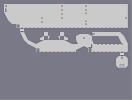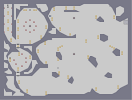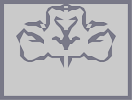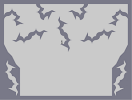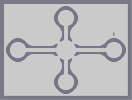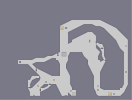thwump run thing 2 Stage Race weird clover type thing waves of thorns Molecular Squeezing Racin' A Rocket In A Tunnel

Pages: (0)

cool

5/5VED

### that's awesome

even the tiles are in the same places upside-down. 5/5 + faved.

Really cool

5/5

great job

### btw

its easier to reverse your screen resolution

### lol

my bad..its rated second art...
yea...im not so sure about that...
dont get me wrong, i really do like it...but second art?..
hmm...not so sure about that...

### McP3000

the longest 5 average was with silverdragon...it had it for around 108 votes if i recal properly...

and before that, my hero was a 5 up to about...80 or so i believe....

no guarantees on the exactness of those numbers...but this levelw as definately not the longest 5...even my arts have had a longer 5 run...(not my much, only about 5)

but this is cool, im not so sure about its top page rating, what is it...5?...but its pretty cool...

### really nice

I like ambigrames.
But I think it isn't an really awesome art.
4/5

### Awww

it was finally attacked
oh well, it was fun and this is still a good ambigrahm

### My god

i think this has been the longest 5/5 average run yet, congratulations.
You've been lucky enough to not encounter any snipers or n-art haters.

### cool

amazing....you did a great work, congratulations

### theres

a picture online that i looked at for how to do it, then did half of it, then did the other half reversed
under google images just look under optical illusions and ull find it, but i didnt use nam i just looked at it

### I noticed what you meant...

and I stand by saying it does, but he said he didn't, so he must not have.

and no NAM.

### 9400

I meant when you load it...should've been more specific.

### hahahaha

that's really amazing.

### Turtle...Yes it does.

But anyways cool, I guess.

### this is obviously a

5/5, I love these ambigrams!! and this is one of the best yet!

i didnt use NAM